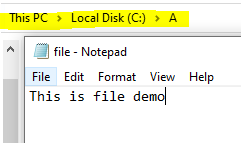Open in App
Not now

# C# Program to Estimate the Size of File Using LINQ

• Last Updated : 01 Nov, 2021

LINQ is known as Language Integrated Query and it is introduced in .NET 3.5. It gives the ability to .NET languages to generate queries to retrieve data from the data source. It removes the mismatch between programming languages and databases and the syntax used to create a query is the same no matter which type of data source is used. In this article, we will estimate the size of the file. So to do this task we use the following methods and class:

1. GetFiles: It will return the name of the files present in a particular directory or subdirectory.

Syntax:

`public static string[] GetFiles (string path);`

where the path is the directory to search. This string is not case-sensitive.

2. Select Method: This method is used to project each element of a sequence into a new form. Or in other words, this method is used when we want to get a single value from the specified collection or sequence.

Syntax:

`Select<TSource,TResult>(IEnumerable<TSource>, Func<TSource,TResult>)`

This is used to select a new File using FileInfo class

3. FileInfo Class: This class provides different types of properties and instance methods that are used in the copying, creation, deletion, moving, and opening of files, and helps in the creation of FileStream objects.

Syntax:

`public sealed class FileInfo : System.IO.FileSystemInfo`

4. Math.Round Method: This method is used to round a value to the nearest integer or to the specified number of fractional digits.

Syntax:

`Math.Round(Decimal, Int32)`

### Approach:

1. Get the file from the path by using the GetFiles() method

`string[] list = Directory.GetFiles("c:\\A\\");`

2. Select the file by using file class and calculate the average using Average() function

`average = list.Select(file =>new FileInfo(file).Length).Average();`

3. Round the size by 1 decimal place using Math.Round Function

`Math.Round(average / 10, 1)`

4. Display the size of the file

Example: In this example, we will find the size of the given file## C#

 `// C# program to estimate the file size ``// Using LINQ``using` `System;``using` `System.Linq;``using` `System.IO;`` ` `class` `GFG{`` ` `static` `void` `Main(``string``[] args)``{``     ` `    ``// Get the file from the path``    ``string``[] list = Directory.GetFiles(``"c:\\A\\"``);``     ` `    ``// Get the average size``    ``var` `average = list.Select(file => ``new` `FileInfo(file).Length).Average();``     ` `    ``// Round off the average size to 1 decimal point``    ``average = Math.Round(average / 10, 1);``     ` `    ``// Display average file size``    ``Console.WriteLine(``"The Average size of the file is {0} MB"``, average);``}``}`

Output:

`The Average size of the file is 1.3 MB`
My Personal Notes arrow_drop_up